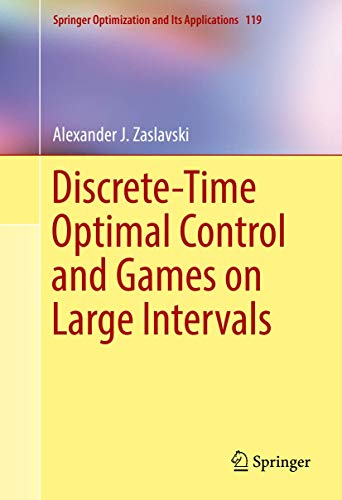# Discrete-Time Optimal Control and Games on Large Intervals: 119 (Springer Optimization and Its Applications)

## Zaslavski, Alexander J.

0 durchschnittliche Bewertung
( 0 Bewertungen bei Goodreads )Alle Exemplare der Ausgabe mit dieser ISBN anzeigen:

<P></P><P></P> <P>DEVOTED TO THE STRUCTURE OF APPROXIMATE SOLUTIONS OF DISCRETE-TIME OPTIMAL CONTROL PROBLEMS AND APPROXIMATE SOLUTIONS OF DYNAMIC DISCRETE-TIME TWO-PLAYER ZERO-SUM GAMES, THIS BOOK PRESENTS RESULTS ON PROPERTIES OF APPROXIMATE SOLUTIONS IN AN INTERVAL THAT IS INDEPENDENT LENGTHWISE, FOR ALL SUFFICIENTLY LARGE INTERVALS. RESULTS CONCERNING THE SO-CALLED TURNPIKE PROPERTY OF OPTIMAL CONTROL PROBLEMS AND ZERO-SUM GAMES IN THE REGIONS CLOSE TO THE ENDPOINTS OF THE TIME INTERVALS ARE THE MAIN FOCUS OF THIS BOOK. THE DESCRIPTION OF THE STRUCTURE OF APPROXIMATE SOLUTIONS ON SUFFICIENTLY LARGE INTERVALS AND ITS STABILITY WILL INTEREST GRADUATE STUDENTS AND MATHEMATICIANS IN OPTIMAL CONTROL AND GAME THEORY, ENGINEERING, AND ECONOMICS.</P> <P>THIS BOOK BEGINS WITH A BRIEF OVERVIEW AND MOVES ON TO ANALYZE THE STRUCTURE OF APPROXIMATE SOLUTIONS OF AUTONOMOUS NONCONCAVE DISCRETE-TIME OPTIMAL CONTROL LAGRANGE PROBLEMS.NEXT THE STRUCTURES OF APPROXIMATE SOLUTIONS OF AUTONOMOUS DISCRETE-TIME OPTIMAL CONTROL PROBLEMS THAT ARE DISCRETE-TIME ANALOGS OF BOLZA PROBLEMS IN CALCULUS OF VARIATIONS ARE STUDIED. THE STRUCTURES OF APPROXIMATE SOLUTIONS OF TWO-PLAYER ZERO-SUM GAMES ARE ANALYZED THROUGH STANDARD CONVEXITY-CONCAVITY ASSUMPTIONS. FINALLY, TURNPIKE PROPERTIES FOR APPROXIMATE SOLUTIONS IN A CLASS OF NONAUTONOMIC DYNAMIC DISCRETE-TIME GAMES WITH CONVEXITY-CONCAVITY ASSUMPTIONS ARE EXAMINED.&NBSP; &NBSP;</P>

Die Inhaltsangabe kann sich auf eine andere Ausgabe dieses Titels beziehen.

Cr�ticas:

"This book is an important contribution to the theory of optimal control and games in the discrete-time framework. The original point of view of the author and the numerous results established provide an innovative approach to these problems which can give food for thought for new researches." (Joel Blot, Mathematical Reviews, February, 2018)

Rese�a del editor:

Devoted to the structure of approximate solutions of discrete-time optimal control problems and approximate solutions of dynamic discrete-time two-player zero-sum games, this book presents results on properties of approximate solutions in an interval that is independent lengthwise, for all sufficiently large intervals. Results concerning the so-called turnpike property of optimal control problems and zero-sum games in the regions close to the endpoints of the time intervals are the main focus of this book. The description of the structure of approximate solutions on sufficiently large intervals and its stability will interest graduate students and mathematicians in optimal control and game theory, engineering, and economics. This book begins with a brief overview and moves on to analyze the structure of approximate solutions of autonomous nonconcave discrete-time optimal control Lagrange problems.Next the structures of approximate solutions of autonomous discrete-time optimal control problems that are discrete-time analogs of Bolza problems in calculus of variations are studied. The structures of approximate solutions of two-player zero-sum games are analyzed through standard convexity-concavity assumptions. Finally, turnpike properties for approximate solutions in a class of nonautonomic dynamic discrete-time games with convexity-concavity assumptions are examined.

��ber diesen Titel� kann sich auf eine andere Ausgabe dieses Titels beziehen.

EUR 70,61

Versand: EUR 28,99
Von Deutschland nach USA

Versandziele, Kosten & Dauer

In den Warenkorb

### Weitere beliebte Ausgaben desselben Titels

#### Vorgestellte Ausgabe

ISBN 10: 3319850199 ISBN 13: 9783319850191
Verlag: Springer, 2018
Softcover## Discrete-Time Optimal Control and Games on Large Intervals

Verlag: Springer-Verlag Gmbh Apr 2017 (2017)
ISBN 10: 3319529315 ISBN 13: 9783319529318
Neu Hardcover Anzahl: 1
Anbieter:
AHA-BUCH GmbH
(Einbeck, Deutschland)

Buchbeschreibung Buch. Zustand: Neu. Neuware -Devoted to the structure of approximate solutions of discrete-time optimal control problems and approximate solutions of dynamic discrete-time two-player zero-sum games, this book presents results on properties of approximate solutions in an interval that is independent lengthwise, for all sufficiently large intervals. Results concerning the so-called turnpike property of optimal control problems and zero-sum games in the regions close to the endpoints of the time intervals are the main focus of this book. The description of the structure of approximate solutions on sufficiently large intervals and its stability will interest graduate students and mathematicians in optimal control and game theory, engineering, and economics.This book begins with a brief overview and moves on to analyze the structure of approximate solutions of autonomous nonconcave discrete-time optimal control Lagrange problems.Next the structures of approximate solutions of autonomous discrete-time optimal control problems that are discrete-time analogs of Bolza problems in calculus of variations are studied. The structures of approximate solutions of two-player zero-sum games are analyzed through standard convexity-concavity assumptions. Finally, turnpike properties for approximate solutions in a class of nonautonomic dynamic discrete-time games with convexity-concavity assumptions are examined. 398 pp. Englisch. Artikel-Nr. 9783319529318

Neu kaufen
EUR 70,61
W�hrung umrechnen
Versand: EUR 28,99
Von Deutschland nach USA
Versandziele, Kosten & Dauer